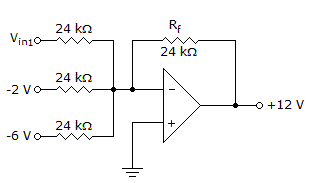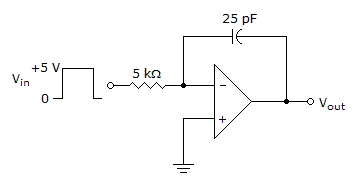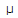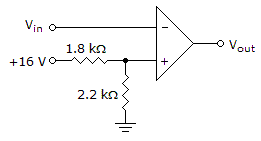# Electronics - Basic Op-Amp Circuits

### Exercise :: Basic Op-Amp Circuits - Filling the Blanks

1.In the given circuit, VIN1 equals ______.

 A. +4 V B. +20 V C. –4 V D. –20 V

Explanation:

No answer description available for this question. Let us discuss.

2.The rate of change of the output voltage in the given circuit equals ______.

 A. 40 mV/s B. 5 V/s C. 0 V/s D. 40 V/s

Explanation:

No answer description available for this question. Let us discuss.

3.

A mathematical operation for finding the area under the curve of a graph is called __________.

 A. differentiation B. integration C. curve averaging D. linear regression

Explanation:

No answer description available for this question. Let us discuss.

4.In the given circuit, if Rf is reduced to 8 k, the circuit becomes the ______.

 A. digital-to-analog converter B. averaging amplifier C. scaling adder D. summing amplifier with gain greater than unity

Explanation:

No answer description available for this question. Let us discuss.

5.The reference voltage for the comparator in the given circuit equals ______.

 A. 0 V B. +8.8 V C. +16 V D. +7.2 V